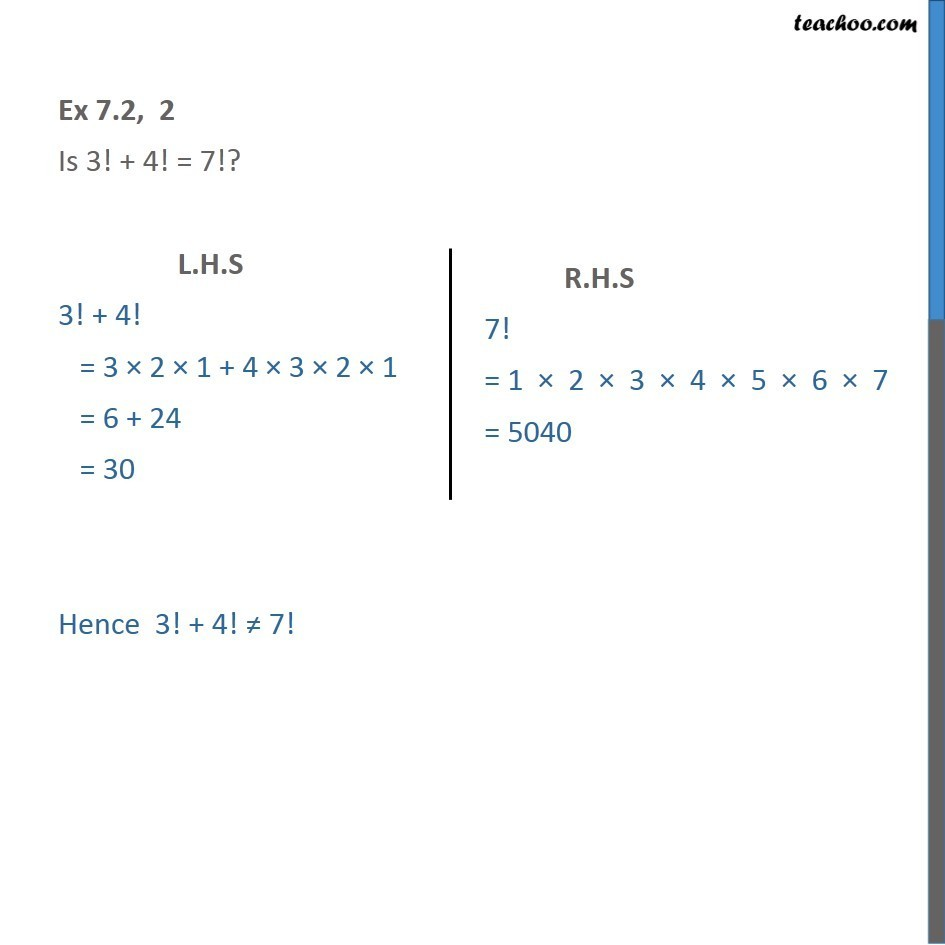1. Chapter 7 Class 11 Permutations and Combinations
2. Serial order wise
3. Ex 7.2

Transcript

Ex 7.2, 2 - Chapter 7 Class 11 Permutations and Combinations - NCERT Is 3! + 4! = 7!? L.H.S 3! + 4! = 3 × 2 × 1 + 4 × 3 × 2 × 1 = 6 + 24 = 30 R.H.S 7! = 1 × 2 × 3 × 4 × 5 × 6 × 7 = 5040 Hence 3! + 4! ≠ 7!

Ex 7.2